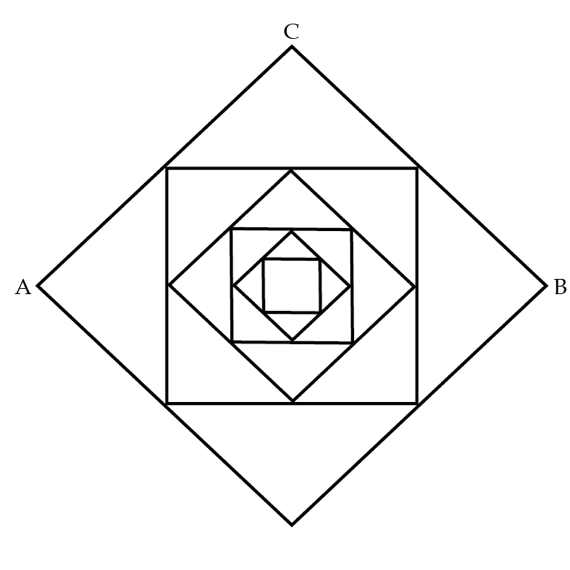# Self-Similar circuit

Consider building a "self-similar infinite" circuit as shown in the figure. Here, the centers of the sides of a square are connected to form another (smaller) square. All the wires are made of the same material and they have equal cross section. What is the equivalent resistance in Ohms between the points A and B if the resistance of the wire AC is $R=1~\Omega$. Note that $R=1~\Omega$ is not the equivalent resistance between A and C, it is just the resistance of the wire AC.×